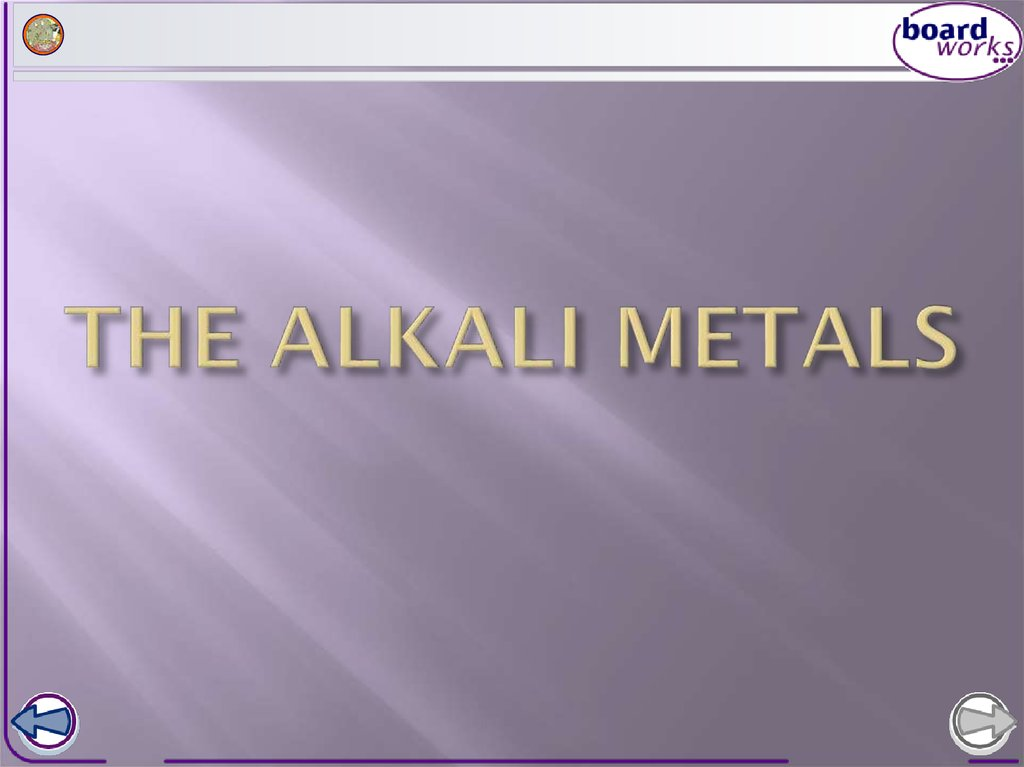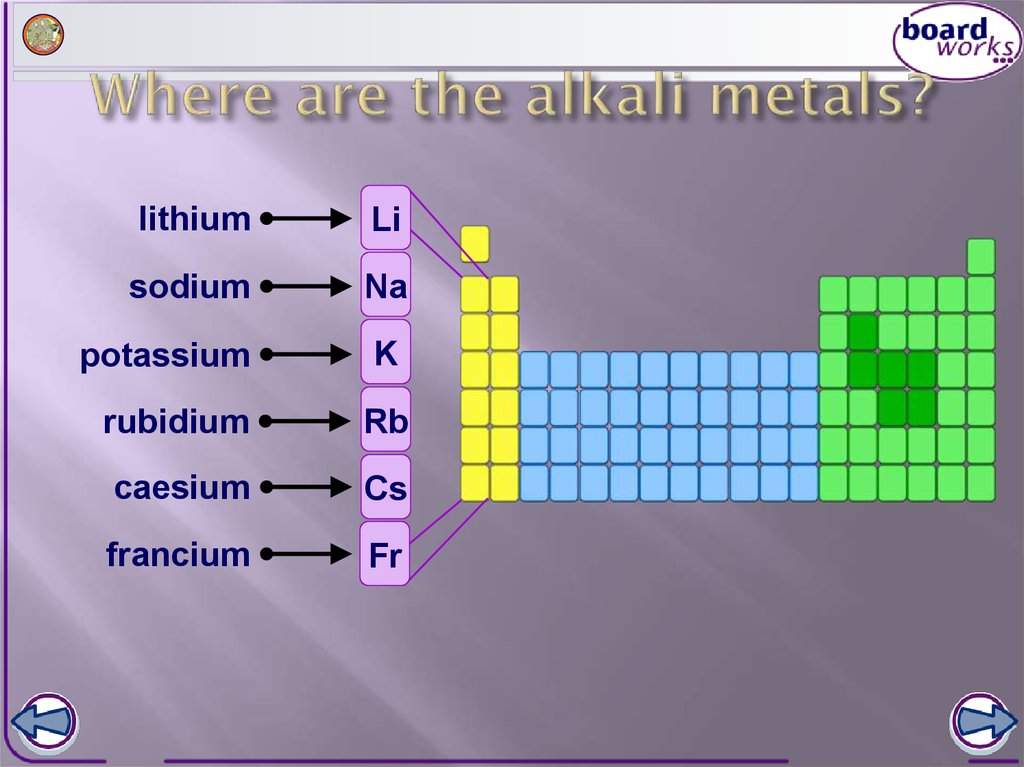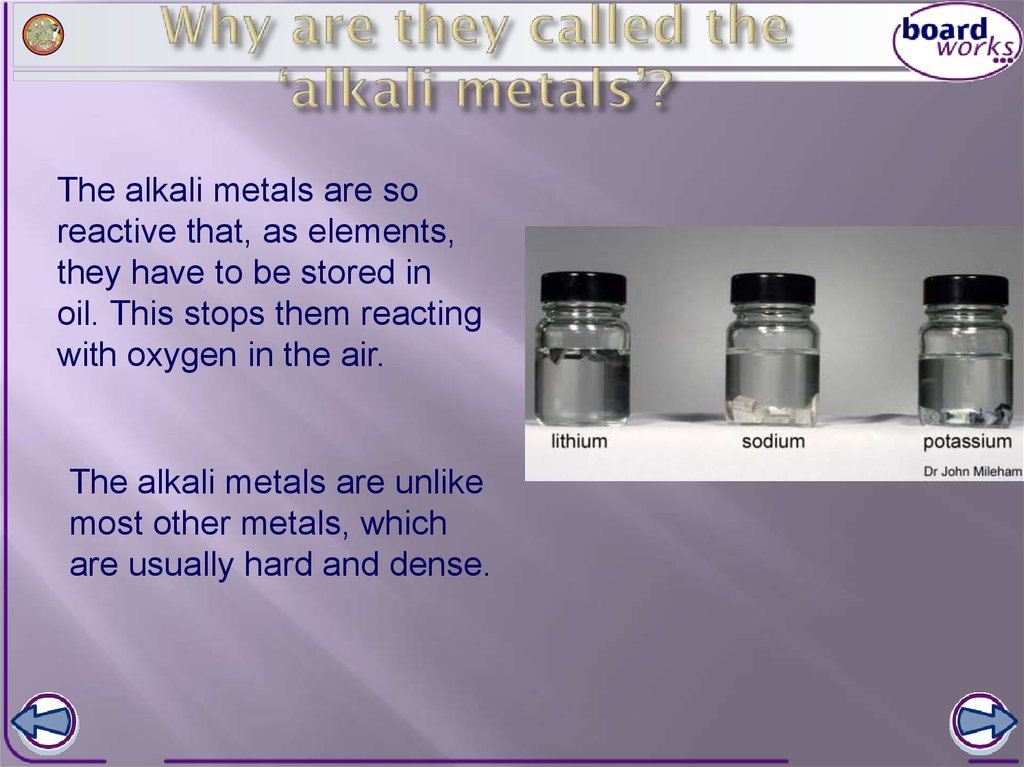# The alkali metals

lithium
Li
sodium
Na
potassium
K
rubidium
Rb
caesium
Cs
francium
Fr

## 3. Why are they called the ‘alkali metals’?

The alkali metals are so
reactive that, as elements,
they have to be stored in
oil. This stops them reacting
with oxygen in the air.
The alkali metals are unlike
most other metals, which
are usually hard and dense.

## 4. What is the electron structure of alkali metals?

The alkali metals all have one electron in their outer shell.
This means that:
They are found in group 1
of the periodic table.
They have similar
physical and chemical
properties.
They can readily lose
the outer shell electron
to form positive ions
with a +1 charge and a
full outer shell.
lithium
2,1
sodium
2,8,1
potassium
2,8,8,1

## 5. What are the properties of the alkali metals?

The characteristic properties of the alkali metals are:
They are soft and can be cut by a knife.
Softness increases going down the group.
They have a low density.
Lithium, sodium and potassium float on water.
They have low melting and boiling points.

## 6. What is the trend in density?

The alkali metals generally become more dense going down
the group, but the trend is not perfect because potassium is
less dense than sodium.
Element
Density (g/dm3)
lithium
0.53
sodium
0.97
potassium
0.86
rubidium
1.53
caesium
1.87

## 7. What is the trend in melting and boiling point?

The melting points and boiling points of alkali metals
decrease going down the group.
Element
Melting point (°C) Boiling point (°C)
lithium
181
1342
sodium
98
883
potassium
64
760
rubidium
caesium
39
28
686
671

## 8. How do the alkali metals react with oxygen?

All alkali metals react
with oxygen in the air
to form metal oxides.
This produces a layer
of dull oxide on the
surface of the metal,
called tarnish.

## 9. What is the equation for the reaction with oxygen?

The reaction between an alkali metal and oxygen is an
example of an oxidation reaction:
alkali metal
+
oxygen alkali metal oxide
The word and chemical equations for the reaction between
lithium and oxygen are:
lithium
4Li (s)
+
+
oxygen lithium oxide
O2 (g)
2Li2O (s)
What are the word and chemical equations for the reaction
that causes sodium to tarnish?
sodium
4Na (s)
+
+
oxygen sodium oxide
O2 (g)
2Na2O (s)

## 11. What does the reaction with water produce?

All the alkali metals react vigorously with
water.
The reaction with water becomes
more vigorous as you go down the
group.
It is an exothermic
reaction as it releases
a lot of heat.

## 12. What is the equation for the reaction with water?

This reaction creates alkaline hydroxide ions.
This is why the group 1 elements are called the alkali metals.
The general equation for the reaction between an alkali
metal reacting with water is:
alkali metal + water
2M(s)
alkali metal + hydrogen
hydroxide
+ 2H2O(l)
2MOH (aq) +
H2(g)

## 13. How does lithium react with water?

Lithium is the least reactive of
the alkali metals.
When added to water, it fizzes
and moves around slowly
across the surface of the water.
What is the equation for
this reaction?
lithium
+
water
lithium
hydroxide
+
hydrogen
2Li (s)
+
2H2O (l)
2LiOH (aq)
+
H2 (g)

## 14. How does sodium react with water?

When added to water, sodium fizzes more than lithium, and
moves quickly across the surface of the water.
The sodium melts as it
reacts, and it becomes
spherical and shiny, like
a ball bearing.
The hydrogen sometimes
catches fire because of
the heat from the reaction.
What is the equation for this reaction?
sodium
+
water
2Na (s)
+
2H2O (l)
sodium
hydroxide
+
hydrogen
2NaOH (aq)
+
H2 (g)

## 15. How does potassium react with water?

When added to water, the
potassium moves across the
surface of the water very quickly.
The reaction produces so much
heat that the hydrogen given off
catches alight.
What colour would the flame be?
Like sodium, it melts with the heat of the reaction.
What is the equation for this reaction?
potassium
+ water potassium + hydrogen
hydroxide
2K (s)
+ 2H2O (l) 2KOH (aq) +
H2 (g)

## 16. How do alkali metals react with water?

Which of the alkali metals will react most strongly with water?

## 17. How does electron structure affect reactivity?

increase in reactivity
The reactivity of alkali metals increases going down the
group. What is the reason for this?
Li
The atoms of each element get larger
going down the group.
Na
This means that the outer shell electron
gets further away from the nucleus and
is shielded by more electron shells.
The further an electron is from the
positive nucleus, the easier it can be
lost in reactions.
K
This is why the reactivity of the alkali
metals increases going down group 1.

## 18. How do alkali metals react with chlorine?

Alkali metals burst into flames when heated and added to
chlorine. They form metal chlorides.
alkali metal
+
chlorine alkali metal chloride
The word and chemical equations for the reaction between
lithium and chlorine are:
lithium
+
2Li (s)
+
chlorine lithium chloride
Cl2 (g)
2LiCl (s)
What are the word and chemical equations for the reaction
that produces sodium chloride?
sodium
+
2Na (s)
+
chlorine sodium chloride
Cl2 (g)
2NaCl (s)